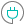#CRITBINOM (PQL - xl)

This function returns the criterion binomial, the smallest value for which the cumulative binomial distribution is greater than or equal to a criterion value

#### Syntax

CRITBINOM(trials, probability s, alpha)

##### Function Arguments
 Name Description Type Optional trials Number of trials; if not an integer, the number is truncated Number probability s Probability of success on each trial; number between 0 and 1 Number alpha Alpha, value for the criterion Number